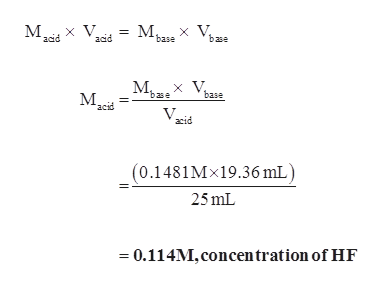# Exactly 19.36 mL of 0.1481 M NaOH is used to titrate a 25.00 mL sample of HF. What is the concentration of the hydrofluoric acid?How many moles of NaOH were used?

Question
1 views

Exactly 19.36 mL of 0.1481 M NaOH is used to titrate a 25.00 mL sample of HF. What is the concentration of the hydrofluoric acid?

How many moles of NaOH were used?

check_circle

Step 1

The balanced chemical equation for the reaction is given below.

Step 2

1 mol HF needs 1 mol NaOH for neutralization.

Number of equivalence of acid = Number of equivalence of base

Macid x Vacid = Mbase x Vbase

And numbe...help_outlineImage TranscriptioncloseV М.х М, V acid base base acid М. хV. base М. base acid acid (0.1481M 19.36 mL) 25 mL =0.114M,concen tration of HF fullscreen

### Want to see the full answer?

See Solution

#### Want to see this answer and more?

Solutions are written by subject experts who are available 24/7. Questions are typically answered within 1 hour.*

See Solution
*Response times may vary by subject and question.
Tagged in

### General Chemistry